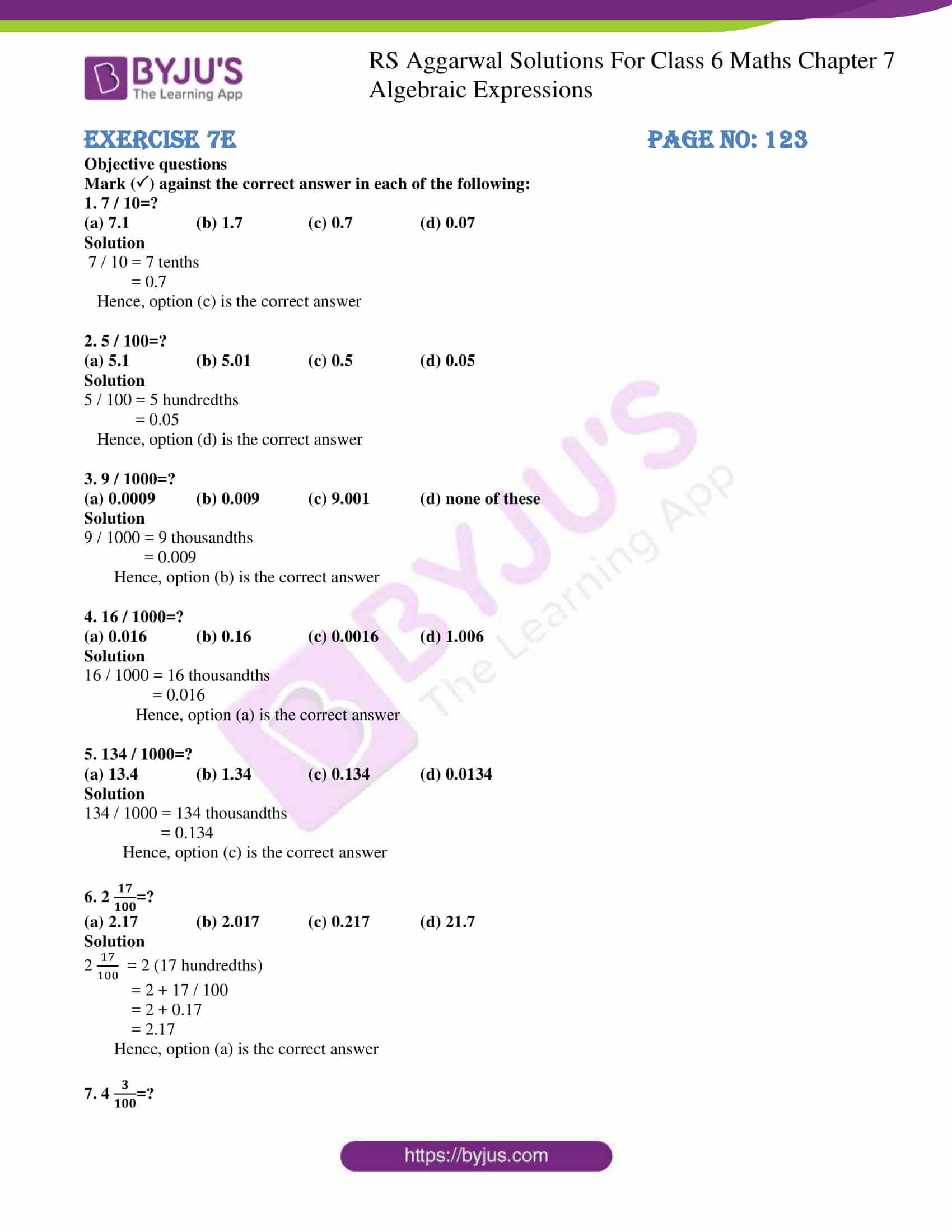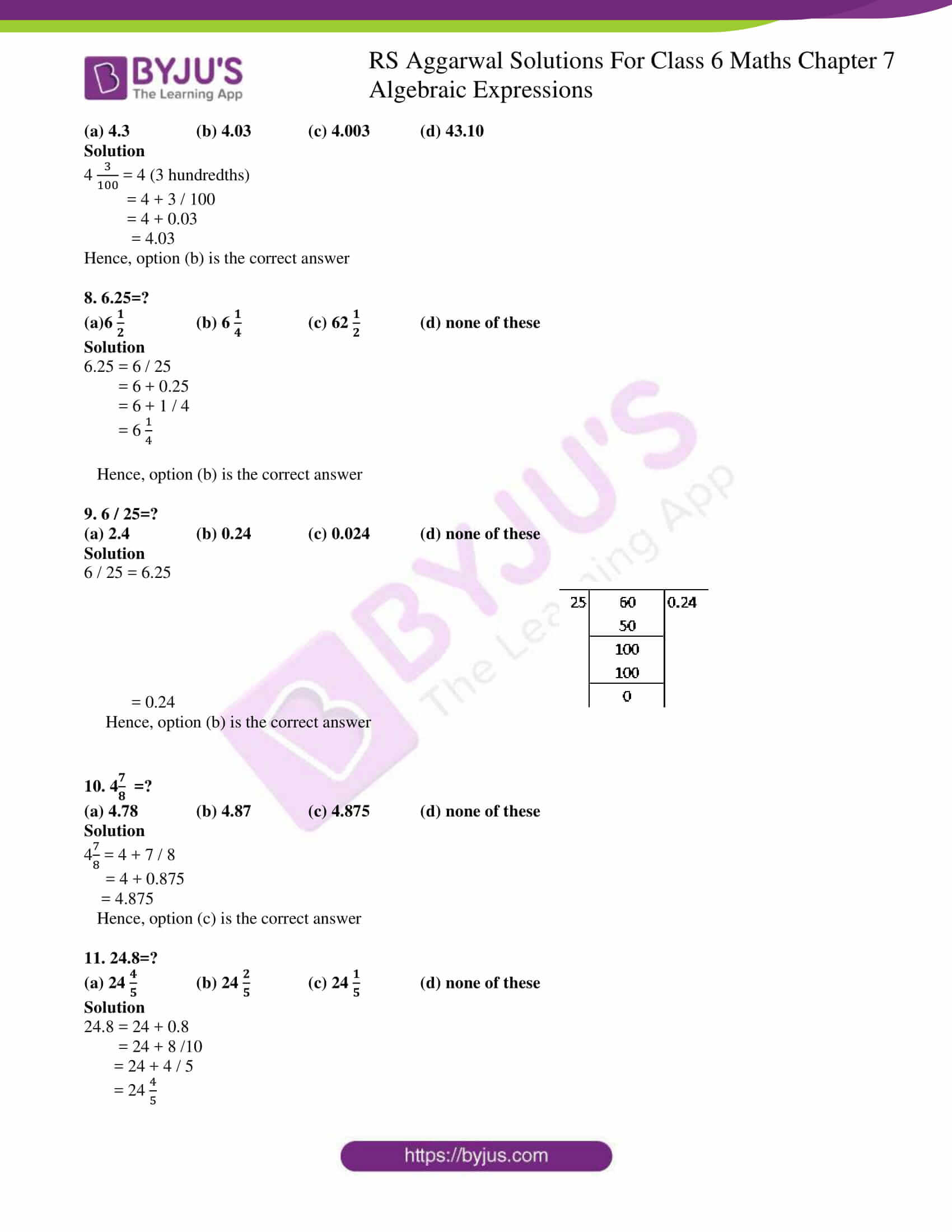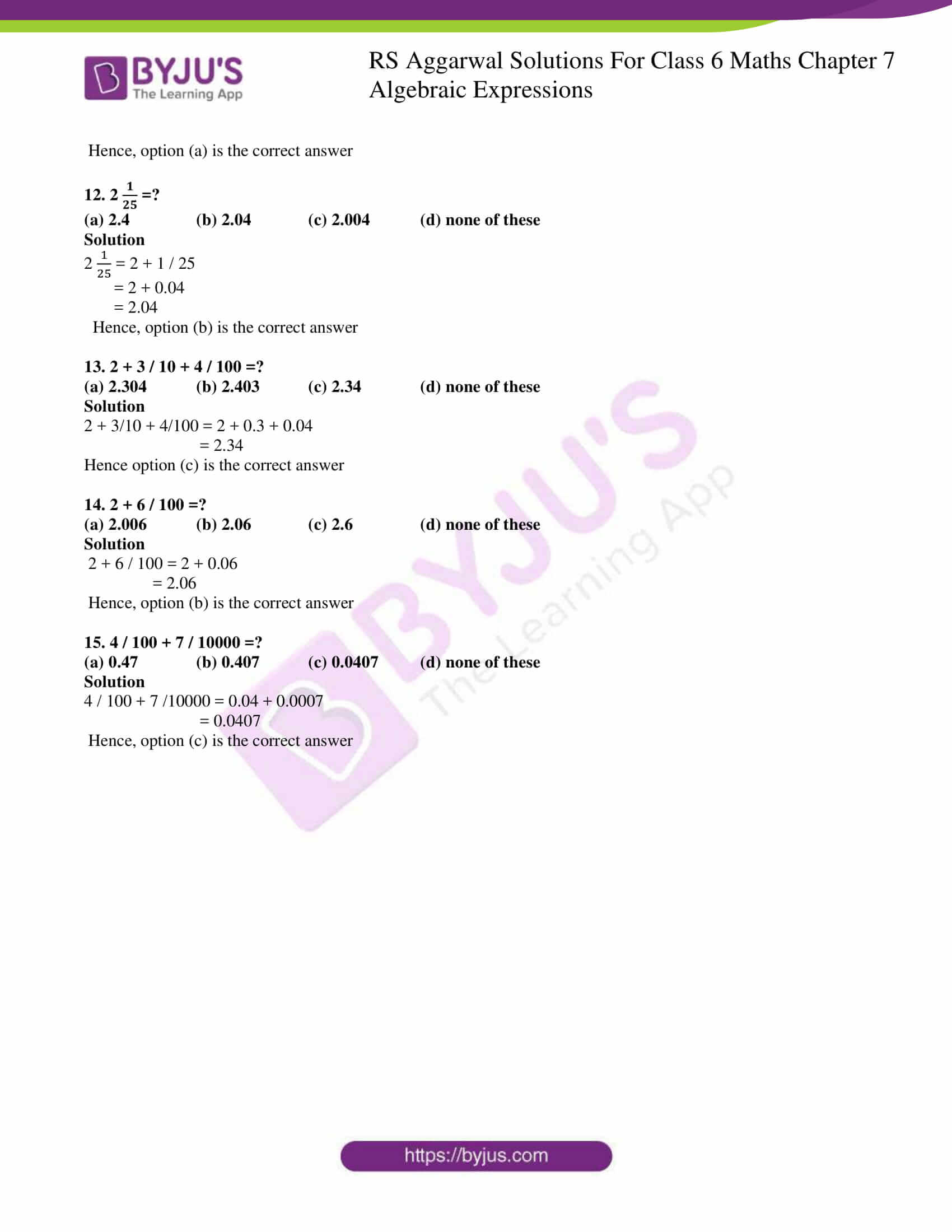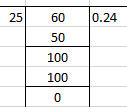# RS Aggarwal Solutions for Class 6 Chapter 7 Decimals Exercise 7E

RS Aggarwal Solutions for Class 6 Chapter 7 Decimals Exercise 7E are available here in PDF format. This exercise consists of objective type questions which covers the concepts of the entire chapter. These questions help the students to check out which concept they have not understood in chapter 17. RS Aggarwal Solutions have been prepared by experts in Mathematics to help students who are struggling to solve problems. Download PDF from their respective links.

## Download PDF of RS Aggarwal Solutions for Class 6 Chapter 7 Decimals Exercise 7E### Access answers to Maths RS Aggarwal Solutions for Class 6 Chapter 7 Decimals Exercise 7E

Objective questions

Mark () against the correct answer in each of the following:

1. 7 / 10=?

(a) 7.1 (b) 1.7 (c) 0.7 (d) 0.07

Solution

7 / 10 = 7 tenths

= 0.7

Hence, option (c) is the correct answer

2. 5 / 100=?

(a) 5.1 (b) 5.01 (c) 0.5 (d) 0.05

Solution

5 / 100 = 5 hundredths

= 0.05

Hence, option (d) is the correct answer

3. 9 / 1000=?

(a) 0.0009 (b) 0.009 (c) 9.001 (d) none of these

Solution

9 / 1000 = 9 thousandths

= 0.009

Hence, option (b) is the correct answer

4. 16 / 1000=?

(a) 0.016 (b) 0.16 (c) 0.0016 (d) 1.006

Solution

16 / 1000 = 16 thousandths

= 0.016

Hence, option (a) is the correct answer

5. 134 / 1000=?

(a) 13.4 (b) 1.34 (c) 0.134 (d) 0.0134

Solution

134 / 1000 = 134 thousandths

= 0.134

Hence, option (c) is the correct answer

6. 2 =?

(a) 2.17 (b) 2.017 (c) 0.217 (d) 21.7

Solution

2 = 2 (17 hundredths)

= 2 + 17 / 100

= 2 + 0.17

= 2.17

Hence, option (a) is the correct answer

7. 4 =?

(a) 4.3 (b) 4.03 (c) 4.003 (d) 43.10

Solution

4 = 4 (3 hundredths)

= 4 + 3 / 100

= 4 + 0.03

= 4.03

Hence, option (b) is the correct answer

8. 6.25=?

(a)6 (b) 6 (c) 62 (d) none of these

Solution

6.25 = 6 / 25

= 6 + 0.25

= 6 + 1 / 4

= 6

Hence, option (b) is the correct answer

9. 6 / 25=?

(a) 2.4 (b) 0.24 (c) 0.024 (d) none of these

Solution

6 / 25 = 6.25

= 0.24Hence, option (b) is the correct answer

10. 4 =?

(a) 4.78 (b) 4.87 (c) 4.875 (d) none of these

Solution

4 = 4 + 7 / 8

= 4 + 0.875

= 4.875

Hence, option (c) is the correct answer

11. 24.8=?

(a) 24 (b) 24 (c) 24 (d) none of these

Solution

24.8 = 24 + 0.8

= 24 + 8 /10

= 24 + 4 / 5

= 24

Hence, option (a) is the correct answer

12. 2 =?

(a) 2.4 (b) 2.04 (c) 2.004 (d) none of these

Solution

2 = 2 + 1 / 25

= 2 + 0.04

= 2.04

Hence, option (b) is the correct answer

13. 2 + 3 / 10 + 4 / 100 =?

(a) 2.304 (b) 2.403 (c) 2.34 (d) none of these

Solution

2 + 3/10 + 4/100 = 2 + 0.3 + 0.04

= 2.34

Hence option (c) is the correct answer

14. 2 + 6 / 100 =?

(a) 2.006 (b) 2.06 (c) 2.6 (d) none of these

Solution

2 + 6 / 100 = 2 + 0.06

= 2.06

Hence, option (b) is the correct answer

15. 4 / 100 + 7 / 10000 =?

(a) 0.47 (b) 0.407 (c) 0.0407 (d) none of these

Solution

4 / 100 + 7 /10000 = 0.04 + 0.0007

= 0.0407

Hence, option (c) is the correct answer